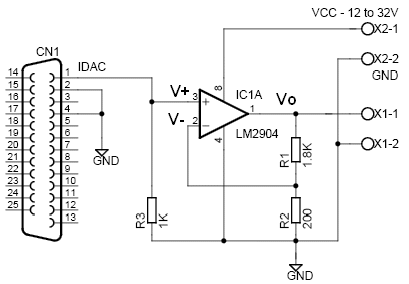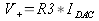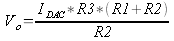# 10V Analog Output Voltage

This application note explains how to obtain a 0 to 10V analog output voltage using the EMANT300. This circuit is used in a proportional valve application. The proportional valve control flow rates from 1.4 to 5m3/h. The variable opening of the cross section is changed proportional to the 0-10V input signal

The analog input range required by the proportional valve is 0 to 10V whereas the analog output of the EMANT300 USB DAQ module is a current from 0 to 1mA (see EMANT300 specs).

The following non inverting opamp circuit is used to convert the 0 to 1mA output to 0 to 10V output.The opamp LM2904 is connected in a negative feedback configuration. V- is given byIDAC is the current output of the EMANT300. V+ is given byIn negative feedback, you can assume that V+ = V- Combining both equations givesSubstituting the component values from the schematic into the equation, gives the following relationshipNote:

1. The supply voltage to the opamp should not exceed 32V for the LM2904.
2. The resistors used should be 1% or better.

## C# code

using System; using System.Collections.Generic; using System.Text; using Emant; namespace Vout { class Vout { static void Main(string[] args) { double volt; string input; Console.WriteLine("Output Voltage 0 - 10"); input = Console.ReadLine(); volt = Convert.ToDouble(input); Emant300 DAQ = new Emant300(); DAQ.Open(); // 10V requires 1mA output current DAQ.WriteAnalog(volt/10); DAQ.Close(); } } }